• r语言折线图
2020-11-20 06:48:54

## 折线图

### 第一部分：概述 & 简单绘图

在R语言中可以使用基本绘图函数lines(x, y, type=)来绘制线条，这里参数x和y分别是数值型向量，代表着横坐标和纵坐标的数据，参数type=主要是用来控制线条的类型

这里需要指出的是lines()函数本身不产生任何图形，通常情况下它只有在plot（）函数后使用才会在图片上添加相关线条。

#在R中分号(;)可以将两个命令连接成一行，如下所示
x <- c(1:5); y <- x # 产生相关数据用于绘图，类似于分别执行x <- c(1:5)和y <- x
par(pch=22, col="red") # 设置绘图符号和颜色，下图表示的就是绘图符号
par(mfrow=c(2,4)) # 设置绘图版面，将画布设置成2行4列的格式，共8个绘图区域
opts = c("p","l","o","b","c","s","S","h") #构建type=类型
for(i in 1:length(opts)){
plot(x, y, type="n", main=heading)
lines(x, y, type=opts[i])
}

# 接下来我们将展示在plot()函数中去除type=这个参数之后的效果
x <- c(1:5); y <- x # create some data
par(pch=22, col="blue") # plotting symbol and color
par(mfrow=c(2,4)) # all plots on one page
opts = c("p","l","o","b","c","s","S","h")
for(i in 1:length(opts)) {
lines(x, y, type=opts[i])
}
# 这里利用for循环来绘制不同区域的图形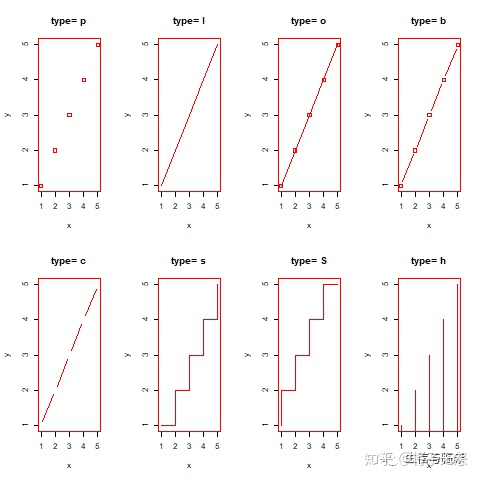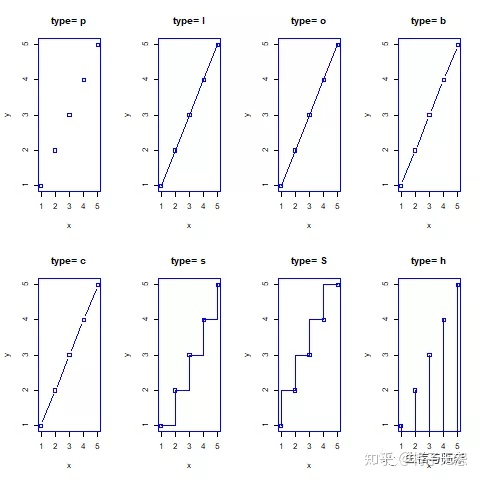从上图中可以看出，只有当绘制点的功能在plot()命令中被抑制时，这个type="c"项才会看起来和type="b"不同。

第二部分：复杂折线图的绘制

接下来我们将利用R语言内置的Orange数据集来展示如何绘制更为复杂的折线图。这里每棵树都会有自己独特的线条。

#首先我们先了解一下Orange（O要大写）数据的基本信息
?Orange

从返回的信息可以看出，该数据集主要有三个参数，一个是Tree，它实际上是树的ID号，从1~5表示共有5棵树，而age表示的是测量时间与

从返回的信息可以看出，该数据集主要有三个参数，一个是Tree，它实际上是树的ID号，从1~5表示共有5棵树，而age表示的是测量时间与1968年12月31日相隔的日数，而circumference则表示树干的周长，所以简单的来看我们可以利用这组数据绘制出每棵树的树干周长随时间变化的趋势。

# 将因子型数据转换成数值型，方便接下来的处理
#推荐读取数据时先设置options(stringsAsFactors=F)
Orange$Tree <- as.numeric(Orange$Tree)
ntrees <- max(Orange$Tree) #获取树的数目 # 获取x轴和y轴的范围 xrange <- range(Orange$age)

## 简介

折线图是用直线将各个数据点连接起来组成的图形。显示数据的变化趋势，一般是随时间的变化。这个图是北方一城市2019年一月份的高温变化。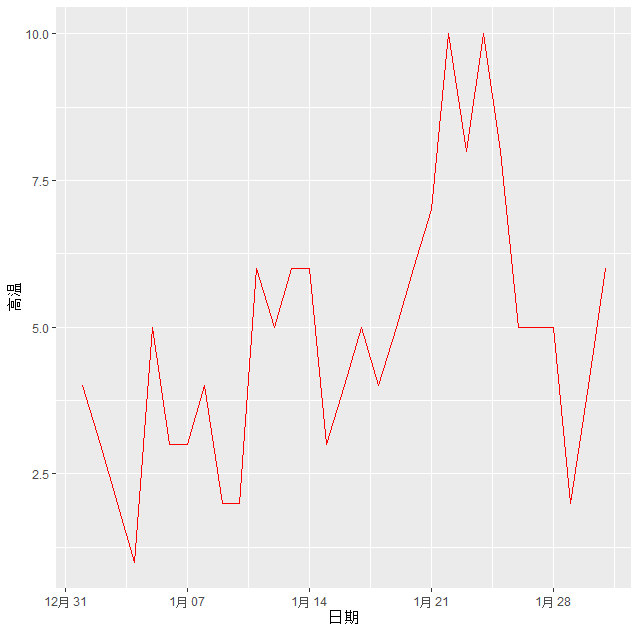## plot

R语言base包里面自带的plotlines可以画折线图。plot可以单独画折线图，lines必须是在其他图的基础之上，单独不可以。
我们先看一个plot的例子。数据还是2019年北方城市一月份的高温。
这个是数据。

        日期 高温 低温
1 2019-01-01    4   -6
2 2019-01-02    3   -7
3 2019-01-03    2   -5
4 2019-01-04    1   -5
5 2019-01-05    5   -6
6 2019-01-06    3   -3

plot(x=tempData$日期,y=tempData$高温,type="l")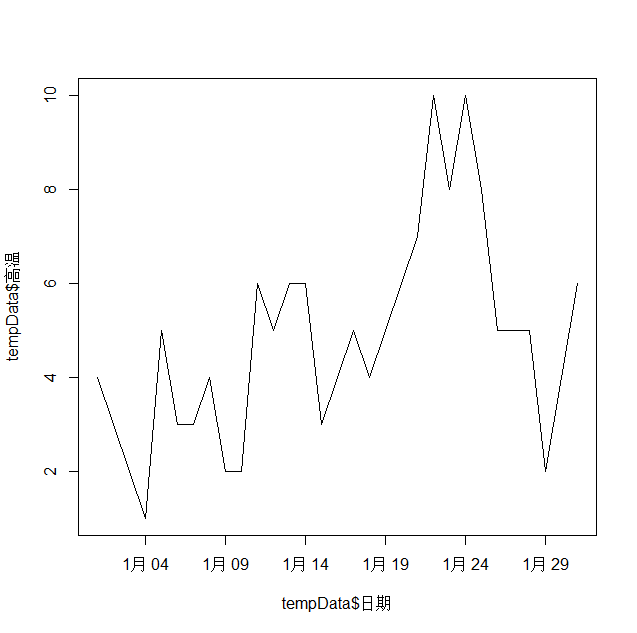plot函数有一个参数type，表示图的类型。默认是p.
“p”，点图。
“l”，线图。
“b”，同时画点和线。
“s”，台阶图。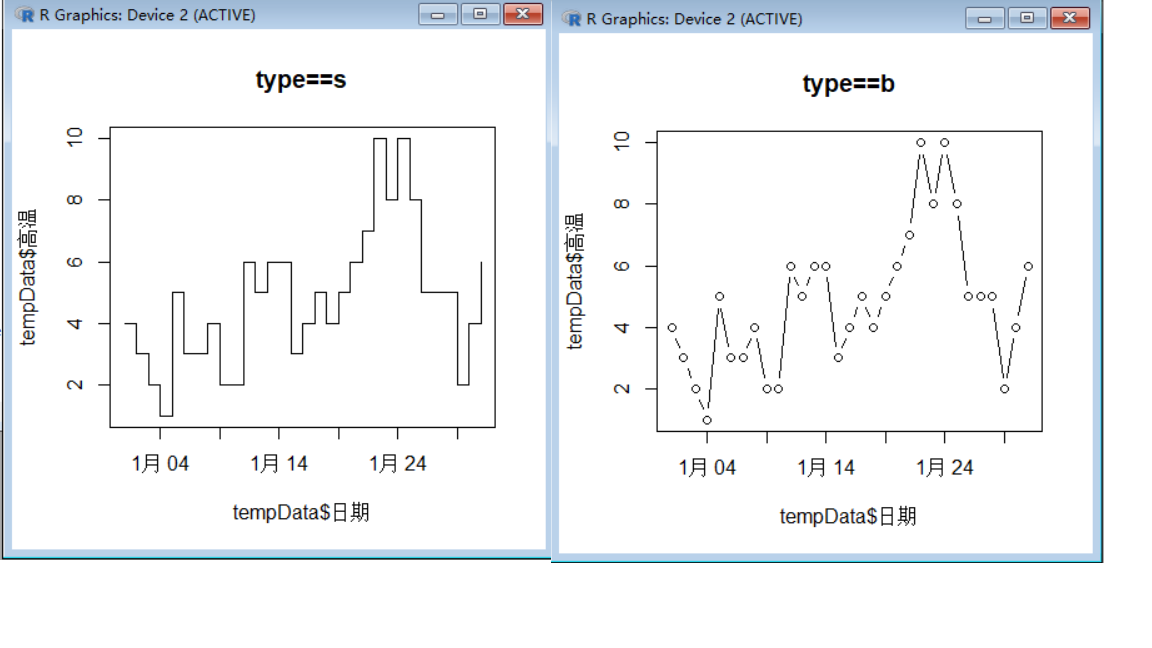## lines

我们在plot折线图的基础上添加一条line。

> head(tempData)
日期 高温 低温
1 2019-01-01    4   -6
2 2019-01-02    3   -7
3 2019-01-03    2   -5
4 2019-01-04    1   -5
5 2019-01-05    5   -6
6 2019-01-06    3   -3
> plot(x=tempData$日期,y=tempData$高温,ylim=c(-10,10),type="l",main="plot with lines",col="red")
> lines(x=tempData$日期,y=tempData$低温,col="blue")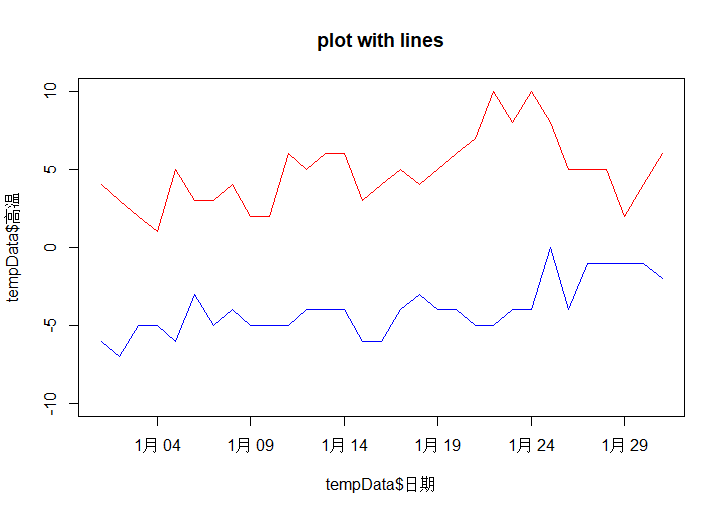## geom_line

这个是我们要用到的数据。我们将用折线图显示2019年，2020年一月份的高温。从图上可以明显的看到2020年一月份的高温基本上都比2019年的高。

> head(newData)
date hightemperature year
1    1               4 2019
2    2               3 2019
3    3               2 2019
4    4               1 2019
5    5               5 2019
6    6               3 2019
> str(newData)
'data.frame':   62 obs. of  3 variables:
$date : int 1 2 3 4 5 6 7 8 9 10 ...$ hightemperature: int  4 3 2 1 5 3 3 4 2 2 ...
$year : Factor w/ 2 levels "2019","2020": 1 1 1 1 1 1 1 1 1 1 ...  library(ggplot2) ggplot(newData, aes(x=date,y=hightemperature,colour = year)) + geom_line()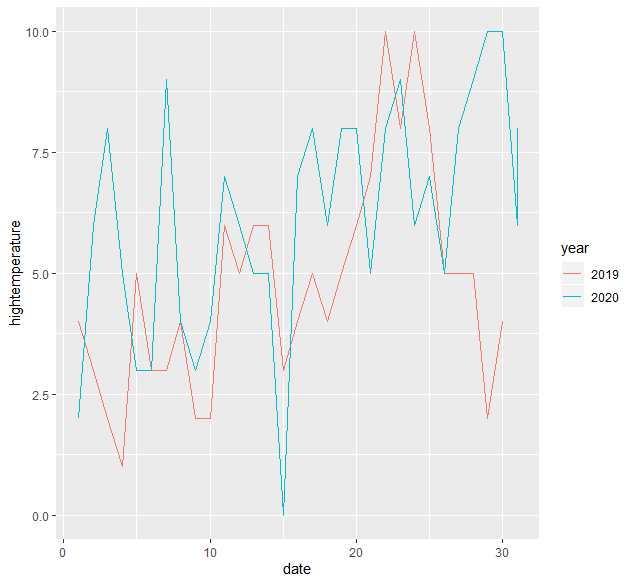我们也可以给上面的图加上点。 ggplot(newData, aes(x=date,y=hightemperature,colour = year)) + geom_line() + geom_point()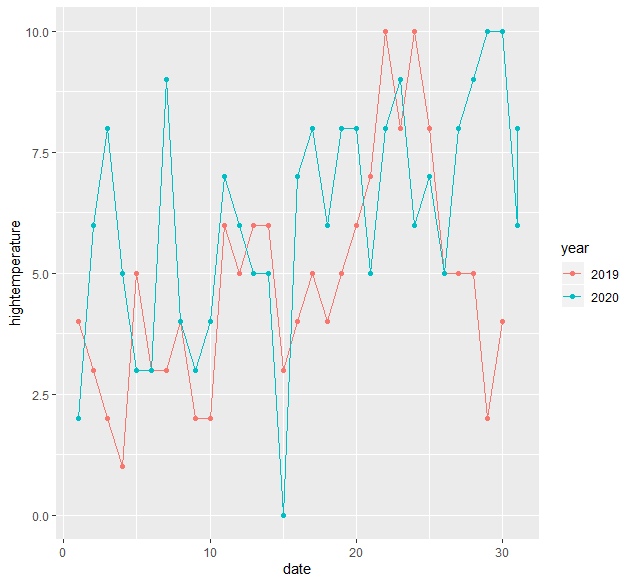geom_line有一个参数叫linetype,表示图上线条的类型，可以设置为数字，也可以设置为string。 0 = blank, 1 = solid, 2 = dashed, 3 = dotted, 4 = dotdash, 5 = longdash, 6 = twodash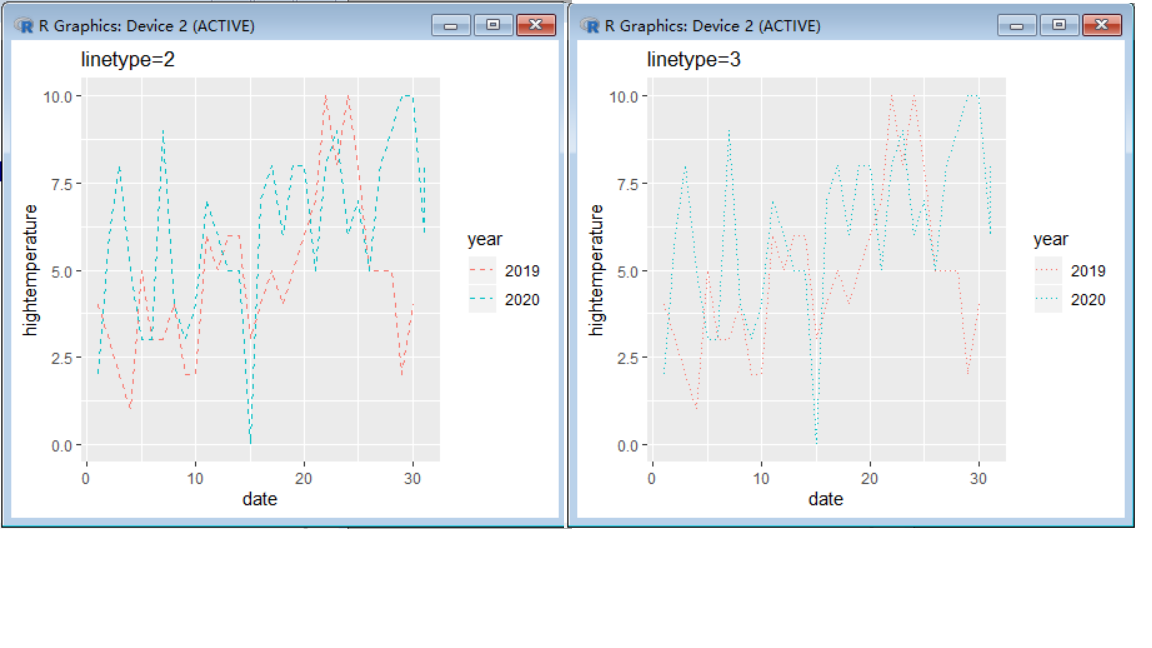展开全文• 折线图简介1. 基础函数2. ggplot()函数3. 实践参考书籍 简介 折线图通常用来对两个连续变量之间的相互依存关系进行可视化。x轴可以是连续型变量，也可以是离散型变量。生物学中，通常用来表示不同药物剂量下实验... ### 折线图 # 简介 折线图通常用来对两个连续变量之间的相互依存关系进行可视化。x轴可以是连续型变量，也可以是离散型变量。生物学中，通常用来表示不同药物剂量下实验对象的变化，或者是基因在不同类型组织或细胞中的表达模式。 # 1. 基础函数 简单示例：使用plot()函数，改变参数type，更多类型请查看帮助文档。 # 查看作图数据 BOD # Time demand # 1 1 8.3 # 2 2 10.3 # 3 3 19.0 # 4 4 16.0 # 5 5 15.6 # 6 7 19.8 op <- par(no.readonly = T) library(dplyr) library(tidyverse) par(mfrow = c(2,2)) BOD %>% { plot(demand ~ Time,data = .,type = "l",main = "A") plot(demand ~ Time,data = .,type = "b",main = "A") plot(demand ~ Time,data = .,type = "s",main = "A") plot(demand ~ Time,data = .,type = "o",main = "A") } par(op)目前，基础函数绘制多个分组折线图，需要借助lines()函数。 op <- par(no.readonly = T) library(dplyr) library(tidyverse) par(mar = c(rep(4,4))) BOD %>% { plot(demand ~ Time,data = .,type = "l",col = "red",lwd = 2) lines(1:7,seq(8,20,length.out = 7),col = "steelblue",lwd = 2) } par(op)多个分组时，可以借助for循环实现。 # 2. ggplot()函数 不加任何参数绘制简单折线图。 library(ggplot2) BOD %>% { ggplot(.,aes(Time,demand))+geom_line() }library(ggplot2) library(patchwork) BOD %>% { p1 <- ggplot(.,aes(Time,demand))+geom_line() p2 <- ggplot(.,aes(factor(Time),demand,group = 1))+geom_line() p1 + p2 }  为了比较因子型和连续型变量的不同，我们将两张图放在一起，可以发现右图中并没有6这个水平。当 x 对应于因子型变量时，必须使用命令 aes(group=1) 以确保 ggplot() 知道这些数据点属于同一个分组，从而应该用一条折线连在一起。相比于基础函数，ggplot绘制分组折线图简直不要太方便。%>%是管道符，需要加载dplyr包，**{}也可以理解为管道符，.**代表上一级生成的数据，p1 + p2 需要加载patchwork拼图包。 library(plyr) ToothGrowth %>% ddply(c("supp", "dose"), summarise, length=mean(len)) %>% { p1 <- ggplot(.,aes(x=dose, y=length, colour=supp)) + geom_line() p2 <- ggplot(.,aes(x=dose, y=length, linetype=supp)) + geom_line() p1 + p2 }如图，分别将supp映射给了颜色和线条类型。 如果要添加数据点等其他类型，可以通过geom系列函数实现。 来吧，实践吧！ # 3. 实践 我使用的是自己的小鼠早期胚胎卵母细胞到8细胞各时期的测序数据，挑选了大约3300个基因。纵坐标使用的是log2(FPKM)值。 一起来看看ggplot绘制分组折线图有多方便吧 首先需要将数据组织成长数据格式。 head(oo1_long)x <- length(unique(oo1_long$t))
ggplot(data=oo1_long, aes(x=variable, y=value, group=t)) +
geom_line(alpha = oo1_long$alpha,color = oo1_long$color,size = oo1_long$size)+ theme_bw()+ scale_y_continuous(expand = c(0,0))+ scale_x_discrete(expand = c(0,0))+ ylab(label = "log2(fpkm)")+ xlab(label = "")+ geom_text(aes(4,10.2,label =paste("cluster1-1", x-1 , sep = '\n')))其实上面的代码还可以再优化，使用aes()函数设置color等参数。 ##侵权请联系作者删除！ # 参考书籍  《R数据可视化手册》 展开全文r语言 • R语言数据可视化包ggplot2画折线图的基本方法r语言 • ## R绘制折线图 千次阅读 2022-02-01 11:07:17 R语言数据分析与可视化从入门到精通》 《R数据可视化手册》 基本图形 R基础函数 使用plot()函数绘制折线图时需向其传递一个包含x值的向量和一 个包含y值的向量，并使用参数type="l"： plot(pressure$temperature...r语言 数据挖掘
• R语言】ggplot2折线图代码 多个时间点折线图 误差线r语言
• R语言使用ggplot2包可视化时间序列折线图、同时可视化多个时间序列的折线图、自定义折线图的颜色数据挖掘 r语言 人工智能 机器学习
• 在使用R语言的ggplot2包绘制折线图时，遇到一个问题，就是当折线太多（我目前要绘制70多组数据的折线），使用线形区分，线形种类不够，使用颜色区分，很多相近的颜色不好分辨，所以考虑到不使用图例，而是在每条折线...r语言
• 论文中折线图可以表示两个变量间的变化关系，带误差或可信区间的折线图表示其中一个变量的变化范围，今天我们通过R语言来演示一下。 继续使用我们的汽车销售数据（公众号回复：汽车销售，可以获得该数据）来演示，...r语言 机器学习 matlab
• 需要下载安装，在Rstudio中用install(“gcookbook”)即可安装，安装后运行程序即可显示相应的折线图。 引言 折线图一般用于描述一维变量随着某一连续变量变化的情况，连续变量通常为时间。换句话
• dose=c(20,30,40,45,60) drugA=c(16,20,27,40,60) drugB=c(15,18,25,31,40) plot(dose,drugA,type="b") ![在这里插入图片描述]... r dose=c(20,30,40,45,60) drugA=c(16,20,27,40,60) drugB=c(15,18,25,31,40).r语言
• R语言ggplot2可视化：可视化折线图、使用labs函数为折线图添加自定义的X轴标签信息r语言 人工智能 数据挖掘 机器学习
• 折线图 数据使用基础安装中的Orange数据集为例，它包含五中橘树的树龄和年轮数据 head(Orange) Grouped Data: circumference ~ age | Tree Tree age circumference 1 1 118 30 2 1 484 58 3 1 664 87...
• R语言ggplot2可视化：可视化折线图、使用labs函数为折线图添加自定义的Y轴标签信息（customize y axis label）r语言 人工智能 数据挖掘 机器学习
• R语言使用rnorm函数生成正太分布数据、使用plot函数可视化折线图、使用legend函数在可视化图像中添加图例信息r语言 机器学习 数据挖掘 人工智能
• xyxminxmaxyminymaxxendyendweightcolor：轮廓色fill：填充色shape：点形状linetype: dotted dashedsize：点大小，线条大小(粗细)alpha：透明度，0-1:完全透明-完全不透明width：宽度(条形等)binwidth：组距(直方...
• 首先建立数据框data，随后调用ggplot函数r语言
• 原标题：在R语言中快速绘制折线图的方法本号前面的文章中介绍了使用abline函数给当前绘图添加直线的方法，本文将介绍在当前绘图中添加直线的另外一个函数：lines函数，同时，使用该函数还可以绘制出类似股票走势图的...
• 原创 小浣熊 科研猫 今天是我们的系统教程《R语言从入门到精通》的第一讲，前面的背景讲解中《从今天开始，每天学点R语言~》，已经深入探讨过R语言的重要性以及学习R语言的必要性，今天我们就按照课表来讲解：如何在...
• 1.什么是柱状图、折线图、面积图？ 柱形图：用于显示一段时间内的数据变化或显示各项之间的比较情况。在柱形图中，类别型或序数型变量映射到横轴的位置，数值型变量映射到矩形的高度。 折线图：用于在连续间隔或...r语言
• 原始数据如下表1，其地址为：C:\\Users\\26869\\Desktop\\r语言.csv。该地址可以用函数file.choose（）选中后运行选择目标文件所获得。读取代码步骤如1： 表1 原始数据 ## supp does len SD SE ...r语言
• ## R语言学习：用ggplot2画折线图

万次阅读 多人点赞 2020-10-12 18:38:24
想把图画做的好看一点，于是学习了一下R语言里的ggplot包。 一、ggplot的通用构造 ggplot(data, aes(x, y)) + gemo_X( ) ggplot(data, aes(x, y)) 这里用来选择使用的数据，以及进行美学处理，aes就是aesthetic的...r语言
• 2.以单个折线图为元素，做出折线图，原始数据如表1，代码如图1： 表1 原始数据 ## supp does len SD SE 1 OJ 1 13.2 4.5 2.111871 2 OJ 2 22.7 3.9 1.977372 ...r语言
• # 绘制条形# 3.1 简单条形# 使用ggplot()函数和geom_bar(stat="identity")library(gcookbook)ggplot(pg_mean,aes(x=group,y=weight))+geom_bar(stat = "identity")# 当x是连续型（数值型）...R语言 条形图 折线图 ggplot2
• R语言ggplot2可视化分面折线图：使用facet_wrap函数可视化分面折线图、使用nrow参数自定义指定分面图中行的个数r语言 数据挖掘 人工智能 机器学习r语言 数据挖掘 机器学习 人工智能
• 29.R语言ggplot2——点图、折线图绘制方法教程.pdf
• 今天我们以R中自带的Orange 数据集为例，来学习折线图的画法，该数据集中包含五种橘树的树龄和年轮数据。要考察橘树的年轮如何随着树龄变化，先画个散点图看看： # 先看第一种橘树，提取第一种树的数据，保存在t1中...r语言折线图...